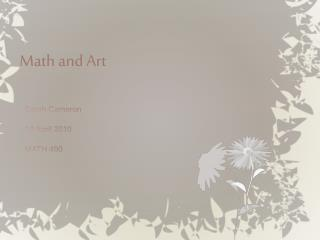# Math and Art - PowerPoint PPT PresentationDownload PresentationMath and Art

Math and ArtDownload Presentation## Math and Art

- - - - - - - - - - - - - - - - - - - - - - - - - - - E N D - - - - - - - - - - - - - - - - - - - - - - - - - - -
##### Presentation Transcript

1. Math and Art Sarah Cameron 12 April 2010 MATH 490

2. outline • Introduction • We will look at math as shown in the art of: • Leonardo da Vinci • Helaman Ferguson • Peter Wang • Discussion

3. Math in art • Math can commonly be seen in art through the use of: • Patterns • Fractals • Tesselations John Biggers, Four Seasons Jock Cooper, Fractal Art

4. Artist: Leonardo davinci • Born April 15, 1452 • Died May 2, 1519 • Educated by Verrocchio • The original Renaissance man • Made significant contributions to: • Art • Math • Science • Engineering

5. Mathematics in Davinci’s art? • Mathematical concepts can be seen in many of da Vinci’s works of art. • Golden rectangles and golden triangles are the most common. "In the Renaissance, you don't find others doing paintings with geometric underlayings to them, whether it's [da Vinci’s] unerring eye . . . or whether it was purposeful, we'll never know.“ – BülentAtalay

6. The Golden Rectangle & triangle • The golden ratio: • 1: 1.618 (approximate) • A golden rectangle is one whose side lengths are in the golden ratio. • A golden triangle is an isosceles triangle in which the two longer sides have equal lengths and in which the ratio of this length to the third, smaller side is the golden ratio.

7. Artist: Helaman fergus0n • Born: August 11, 1940 • Most of his works are sculpture. • Was raised by a distant cousin and her husband in Palmyra, New York. • His 9th grade math teacher recognized Ferguson’s talent for both math and art. • Assigned him projects such as creating a wire-frame model of a hyperbolic paraboloid. • Was a math professor at BYU from 1972-1988

8. Ferguson’s math-sculpture • Ferguson is very purposeful about creating mathematical art. • Most of his sculptures are related to topology. Whaledream II “I find that sculpture is a very powerful way to convey mathematics, and mathematics is a very powerful design language for sculpture.” -Helaman Ferguson Torus with Cross-Cap and Vector Field

9. Topology • The branch of mathematics that deals with the fundamental characteristics of geometric shapes that remain the same even when the shape is twisted, stretched, or otherwise distorted, as long as the transformation doesn’t involve tearing or breaking. • Objects studied in topology include: • Mobius Strip • Torus

10. Double Torus Stonehenge • 28 individual sculptures arranged in a circle. • Each figure is a representation of a double torus. • The figures depict in 28 stages how the two handles of a double torus can link and unlink without breaking or tearing. • “In effect, [the sculpture], is both a theorem and its proof.”

11. Four Canoes • Each ring represents a Klein surface. • Only has one side • Can be represented as a “bottle” or “figure-eight” • The tiled floor that Four Canoes rests on is a 2-D way to visualize a Klein surface. • Whether the Klein tessellation can be extended to infinity has not yet been proved.

12. Artist: Peter Wang • Graduated from CalTech in 1998 with a B.S. in Mathematics • Then took a ceramics course at Pasadena City College, and began a career as a potter • Wang is known for his double-walled creations.

13. How he creates double walled bowls • He draws preliminary sketches on graph paper. • From the initial sketches he derives a series of equations that describe the lines and shapes. • Those equations are entered into Mathematica, where they are plotted to fit the shape of the desired vessel. • The design is transferred to the vessel by pressing map tacks through the paper into the clay. • The design is then carved out of the clay with an x-acto knife.

14. Phyllotaxis Spiral • In botany, phyllotaxis is the arrangement of leaves on a stem or axis. • Spiral is just one type of phyllotaxis. • It can be represented in polar coordinates by the following: • r = c √n, Θ = n ∙ 137.5º • Θ is the angle, • r is the radius • n is the index number of the floret • c is a constant scaling factor • It is a form of Fermat’s Spiral. • The number of left and right spirals are successive Fibonacci numbers.

15. The Golden Angle • The golden angle is the smaller of the two angles that are created by dividing the circumference of a circle into two arcs where the lengths are in proportion equal to the golden ratio. • It is equal to approximately 137.5º or 2.399 radians

16. Discussion • Can you think of a piece of artwork that has reminded you of a mathematical topic? • Does learning about math in the context of art help you understand either the math or the art better?